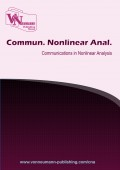# Nash equilibrium strategy for two-person zero-sum matrix games on credibility space

Volume 5, Issue 1, pp 1--7 Publication Date: 2018-04-29### Authors

Fatemeh Madandar - Department of Mathematics, Karaj Branch, Islamic Azad University, Karaj, Iran
Somayeh Haghayeghi - Department of Mathematics, Karaj Branch, Islamic Azad University, Karaj, Iran

### Abstract

In this paper, firstly, we obtain the credibility measure of fuzzy trapezoidal variables. Also, we attain the expected value of fuzzy trapezoidal variables. Then, based on these theorems, we present the expected value Nash equilibrium strategy of the fuzzy games. In other words we extend the expected model to fuzzy trapezoidal variables and improve the previous researches in this area. However in some cases, the game don't have the Nash equilibrium strategy. Therefore, we investigate the existence of Pareto Nash equilibrium and weak Pareto Nash equilibrium strategies in this cases.

### Keywords

Matrix game, fuzzy payoffs, Nash equilibrium, fuzzy trapezoidal variables

### References

•  L. Baoding, L. Yian-Kui, Expected value of fuzzy variable and fuzzy expected value models,, IEEE Transactions on Fuzzy Systems, 10 (2002), 445-450

•  B. Dutta, S. K. Gupta, On Nash equilibrium strategy of two-person zero-sum games with trapezoidal fuzzy payoffs, Fuzzy Inf. Eng., 6 (2014), 299-314

•  L. Cunlin, Z. Qiang, Nash equilibrium strategy for fuzzy non-cooperative games, Fuzzy Sets and Systems, 176 (2011), 46-55